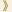HomeShaw Laureates2013Mathematical SciencesEssay

For more than two decades David Donoho has been a leading figure in mathematical statistics. His introduction of novel mathematical tools and ideas has helped shape both the theoretical and applied sides of modern statistics. His work is characterized by the development of fast computational algorithms together with rigorous mathematical analysis for a wide range of statistical and engineering problems.

A central problem in statistics is to devise optimal and efficient methods for estimating (possibly non-smooth) functions based on observed data which has been polluted by (often unknown) noise. Optimality here means that, as the sample size increases, the error in the estimation should decrease as fast as that for an optimal interpolation of the underlying function. The widely used least square regression method is known to be non-optimal for many classes of functions and noise that are encountered in important applications, for example non-smooth functions and non-Gaussian noise. Together with Iain Johnstone, Donoho developed provably almost optimal (that is, up to a factor of a power of the logarithm of the sample size) algorithms for function estimation in wavelet bases. Their “soft thresholding” algorithm is now one of the most widely used algorithms in statistical applications.

A key theme in Donoho’s research is the recognition and exploitation of the fundamental role of sparsity in function estimation from high dimensional noisy data. Sparsity here refers to a special property of functions that can be represented by only a small number of appropriately chosen basis vectors. One way to characterize such sparsity is to minimize the L0-norm of the coefficients in such representations. Unfortunately, the L0-norm is not convex and is highly non-smooth, making it difficult to develop fast algorithms for its computation. In addition to pioneering the exploitation of sparsity, Donoho also introduced the computational framework for using the L1-norm as a convexification of the L0-norm. This has led to an explosion of efficient computational algorithms realizing this sparsity framework which have been used effectively in a wide variety of applications, including image processing, medical imaging, data mining and data completion.

A recent and much celebrated development along this sparsity–L1 theme is Compressed Sensing (a term coined by Donoho). Data compression is widely used nowadays — for example the JPEG standard for compressing image data. Typically, the data is gathered from sensors (for example a camera) and the data is then compressed (that is represented by a much smaller number of coefficients in an appropriate basis, while preserving as much accuracy as possible). Corresponding de-compression algorithms are then used to recover the original data. The revolutionary idea in Compressed Sensing is to shortcut this standard approach and to “compress while sensing”, that is to collect a small number of appropriately chosen samples of the data, from which the original data can be recovered (provably exactly under appropriate assumptions) through corresponding de-compression algorithms. The key ingredients are again sparsity (most typical in a wavelet basis), use of L1-norm for recovery, and the use of random averaging in sensing. Along with Emmanuel Candes and Terence Tao, Donoho is widely credited as one of the pioneers of this exploding area of research, having contributed fundamental ideas, theoretical frameworks, efficient computational algorithms and novel applications. This is still a thriving area of research with wide applications, but already many stunning results have been obtained (both theoretical and practical).

Mathematical Sciences Selection Committee
The Shaw Prize

23 September 2013   Hong Kong